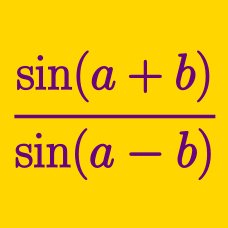Geometry

# Double Angle Identities

Given that $$\sin^2 \theta = \frac{1}{3}$$, what is the value of $$72 \cos(2 \theta)$$?

It is given that $$\sin(\theta) = \frac{1}{\sqrt{37}}$$. If $$\cos ( 2 \theta ) = \frac{a}{b}$$, where $$a$$ and $$b$$ are coprime positive integers, what is the value of $$a+b$$?

$$x$$ is an angle measured in radians and satisfies $$\frac{-\pi}{2} < x < \frac{\pi}{2}$$. It is given that $$\sin(x) = \frac{8}{17}$$. If $$\sin(2x)$$ can be written as $$\frac{a}{b}$$, where $$a$$ and $$b$$ are positive, coprime integers, what is the value of $$a+b$$?

The double angle identity states that

$\cos (2 \theta) = N \cos^2 \theta - M,$

where $$N$$ and $$M$$ are real numbers. What is the value of $$N + M$$?

The double angle identity states that

$\sin ( 2 \theta ) = N \sin \theta \cos \theta + M,$

where $$N$$ and $$M$$ are real numbers. What is the value of $$N + M$$?

×